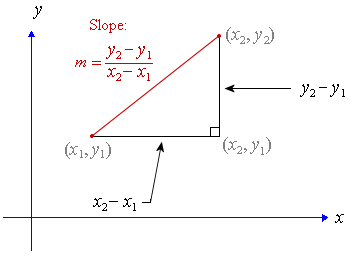top of page
Search

# How to find gradient of a straight line

Updated: Jun 16, 2021

Dear students,

Math Lobby will be teaching you how to find the gradient of a straight line.

Finding gradient of a straight line is covered under the topic Coordinate Geometry in Secondary 2 Math, Secondary 3 Express E Math and A Math, Secondary 3 Normal Math and A math.Firstly, we have to understand the term gradient of a line. Gradient of a line represents the steepness of a line. From this, we can infer that the larger the gradient, the steeper the line.

Secondly, we have to understand the terms in the gradient formula: (Y2-Y1)/(X2-X1)

X1: X coordinate of the 1st point

X2: X coordinate of the 2nd point

Y1: Y coordinate of the 1st point

Y2: Y coordinate of the 2nd point

Lastly, we will learn how to apply the gradient formula to find the gradient of a straight line.

Example:

Find the gradient of the line connecting points A and B where point A is (2,4) and point B is (6,10)

= (10-4)/(6-2)

= 6/4

= 3/2

If you want to receive daily Secondary Math Tips,

FOLLOW our Instagram page at https://www.instagram.com/mathlobbymotivation/

*

*

*

(Image Credit: Intmath)